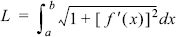# Numerical Integration

Core Topics

Rectangle and midpoint methods (9.2).

Trapezoidal method (9.3).

Simpson's methods (9.4).

Evaluation of multiple integrals (9.6).

Use of MATLAB built-in functions for integration (9.7).

Complementary Topics

Estimation of error (9.8).

Richardson's extrapolation (9.9).

Romberg integration (9.10).

Improper integrals (9.11).

## 9.1 BACKGROUND

Integration is frequently encountered when solving problems and calculating quantities in engineering and science. Integration and integrals are also used when solving differential equations. One of the simplest examples for the application of integration is the calculation of the length of a curve (Fig. 9-1). When a curve in the x-y plane is given by the equation y = f(x), the length L of the curve between the points x = a and x = b is given by:Figure 9-1: Length of a curve.

In engineering there are circumstances that involve experimental or test data, where a physical quantity that has to be determined may be expressed as an integral of other quantities that are measured. For example, the total rate of heat flow through a cross section of width W and height (ba) is related to the local heat flux via an integral (see Fig. 9-2):

Figure 9-2: Heat flux through a rectangular cross ...

Get Numerical Methods for Engineers and Scientists 3rd Edition now with the O’Reilly learning platform.

O’Reilly members experience books, live events, courses curated by job role, and more from O’Reilly and nearly 200 top publishers.